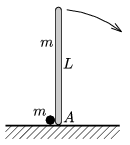Mathematical and Physical Journal
for High Schools
Issued by the MATFUND Foundation
 Already signed up? New to KöMaL?

#Problem P. 4330. (March 2011)

P. 4330. A thin, L=2 m long rod of mass m is standing vertically on a horizontal frictionless plane. Next to its bottom endpoint A there is a pointlike object of mass m at rest. The rod fells out of its unstable equilibrium position, such that the paths of the small body and the rod are always in the same plane. What is the greatest speed of the small body?(6 pont)

Deadline expired on April 11, 2011.

Sorry, the solution is available only in Hungarian. Google translation

Megoldás. Ha a pálca $\displaystyle \theta$ szöget zár be a függőlegessel, a kis test sebessége

$\displaystyle v=\sqrt{gL\frac{\cos^2\theta-\cos^3\theta}{\frac{16}3-2\cos^2\theta}}\le 0{,}82~\frac{\rm m}{\rm s}.$

### Statistics:

 28 students sent a solution. 6 points: Béres Bertold, Kovács 444 Áron, Laczkó Zoltán Balázs, Nagy Lajos, Papp Roland, Szabó 928 Attila, Szigeti Bertalan György, Ürge László, Várnai Péter. 5 points: Bolgár Dániel, Nagy Dániel Bálint, Sárvári Péter, Szélig Áron. 4 points: 2 students. 3 points: 4 students. 2 points: 4 students. 1 point: 3 students. 0 point: 2 students.

Problems in Physics of KöMaL, March 2011